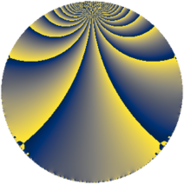# Properties

 Label 441.4.bbLevel $441$ Weight $4$ Character orbit 441.bb Rep. character $\chi_{441}(37,\cdot)$ Character field $\Q(\zeta_{21})$ Dimension $828$ Sturm bound $224$

# Related objects

## Defining parameters

 Level: $$N$$ $$=$$ $$441 = 3^{2} \cdot 7^{2}$$ Weight: $$k$$ $$=$$ $$4$$ Character orbit: $$[\chi]$$ $$=$$ 441.bb (of order $$21$$ and degree $$12$$) Character conductor: $$\operatorname{cond}(\chi)$$ $$=$$ $$49$$ Character field: $$\Q(\zeta_{21})$$ Sturm bound: $$224$$

## Dimensions

The following table gives the dimensions of various subspaces of $$M_{4}(441, [\chi])$$.

Total New Old
Modular forms 2064 852 1212
Cusp forms 1968 828 1140
Eisenstein series 96 24 72

## Trace form

 $$828q + 13q^{2} + 259q^{4} - q^{5} - 10q^{7} + 68q^{8} + O(q^{10})$$ $$828q + 13q^{2} + 259q^{4} - q^{5} - 10q^{7} + 68q^{8} - 24q^{10} + 111q^{11} - 186q^{13} - 39q^{14} + 1023q^{16} + 127q^{17} - 486q^{19} + 382q^{20} - 754q^{22} + 321q^{23} + 1794q^{25} + 108q^{26} + 304q^{28} - 228q^{29} - 1290q^{31} - 1659q^{32} + 402q^{34} + 1113q^{35} - 2001q^{37} - 1526q^{38} + 2110q^{40} + 1698q^{41} + 270q^{43} + 5390q^{44} + 3828q^{46} + 1521q^{47} - 2240q^{49} - 6052q^{50} - 3882q^{52} - 1037q^{53} - 3790q^{55} - 1928q^{56} - 12q^{58} - 827q^{59} - 445q^{61} + 430q^{62} - 10428q^{64} + 364q^{65} + 834q^{67} + 3607q^{68} - 8398q^{70} - 3112q^{71} + 629q^{73} + 1604q^{74} - 445q^{76} - 355q^{77} + 848q^{79} + 3182q^{80} - 13890q^{82} - 3258q^{83} - 176q^{85} - 471q^{86} + 5545q^{88} - 6529q^{89} + 8280q^{91} - 7966q^{92} + 9814q^{94} + 9328q^{95} + 4052q^{97} + 13415q^{98} + O(q^{100})$$

## Decomposition of $$S_{4}^{\mathrm{new}}(441, [\chi])$$ into newform subspaces

The newforms in this space have not yet been added to the LMFDB.

## Decomposition of $$S_{4}^{\mathrm{old}}(441, [\chi])$$ into lower level spaces

$$S_{4}^{\mathrm{old}}(441, [\chi]) \cong$$ $$S_{4}^{\mathrm{new}}(49, [\chi])$$$$^{\oplus 3}$$$$\oplus$$$$S_{4}^{\mathrm{new}}(147, [\chi])$$$$^{\oplus 2}$$